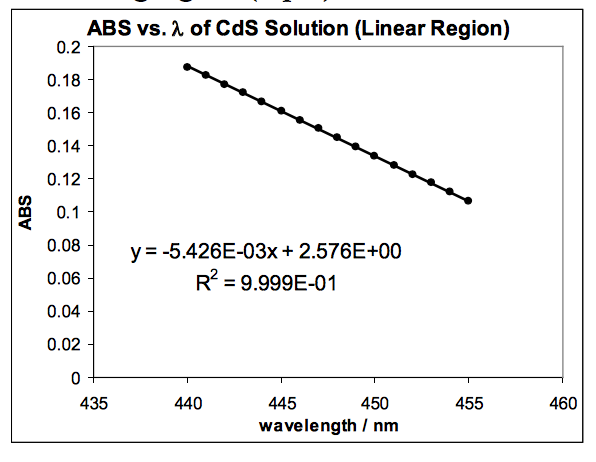# CdS NanoparticlesThere are 3 parts that are all related to each other, but I'm not sure where to start:

• The graph below is the linear portion from the graph above. What is the x-intercept (with units) for the line below? What does this value represent, besides the wavelength at which particles stop absorbing light?
• What is the energy (in Joules) corresponding to the wavelength determined in previous question, ~474.8 nm
• Calculate the particle radius using the equation in the lab packet and the energy calculated in latest question (Q2)